# DIY Si4730 All Band Radio (LW, MW, SW, FM)

IntermediateFull instructions provided55,279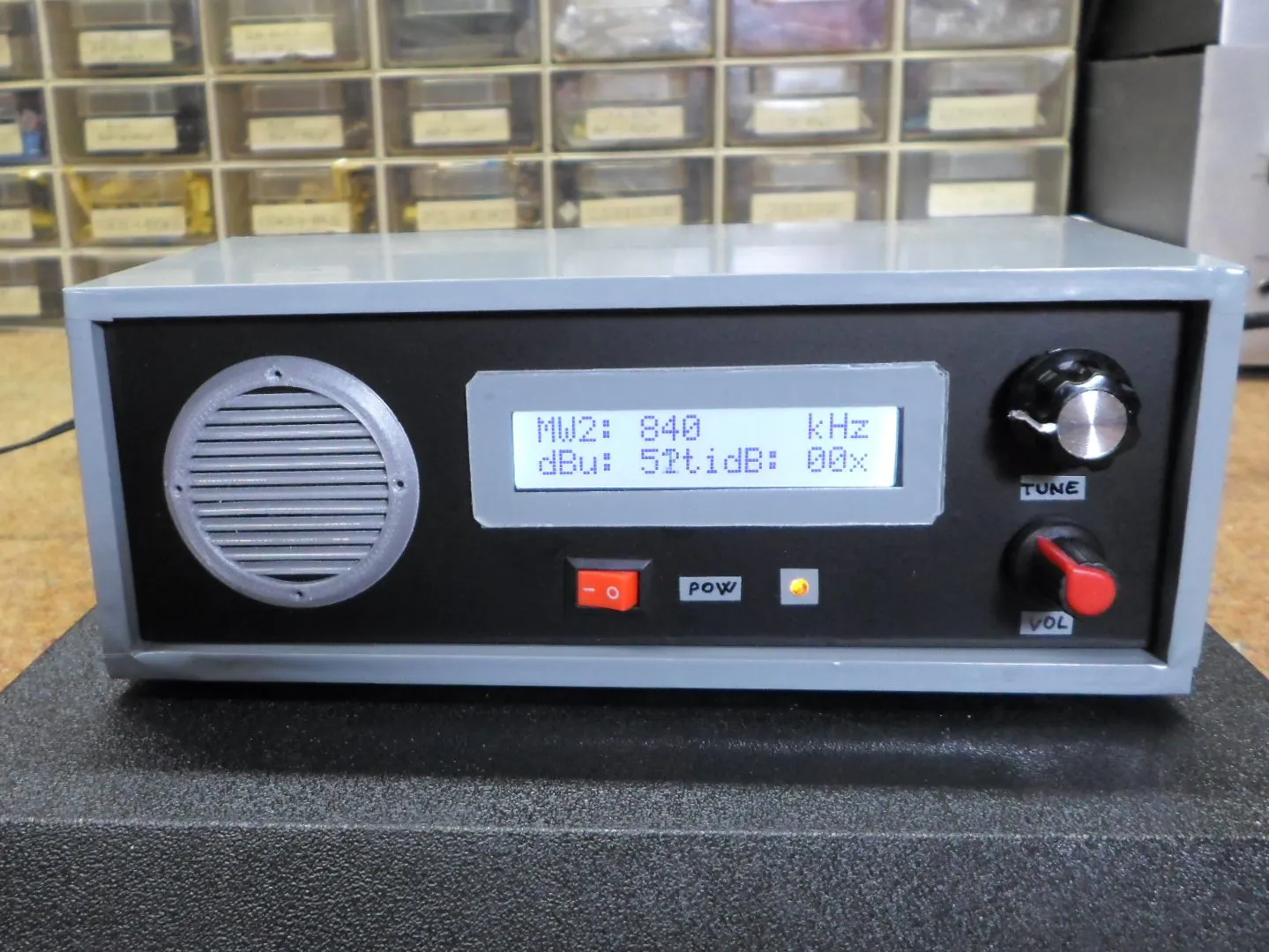## Things used in this project

### Hardware componentsArduino Nano R3
×1
×1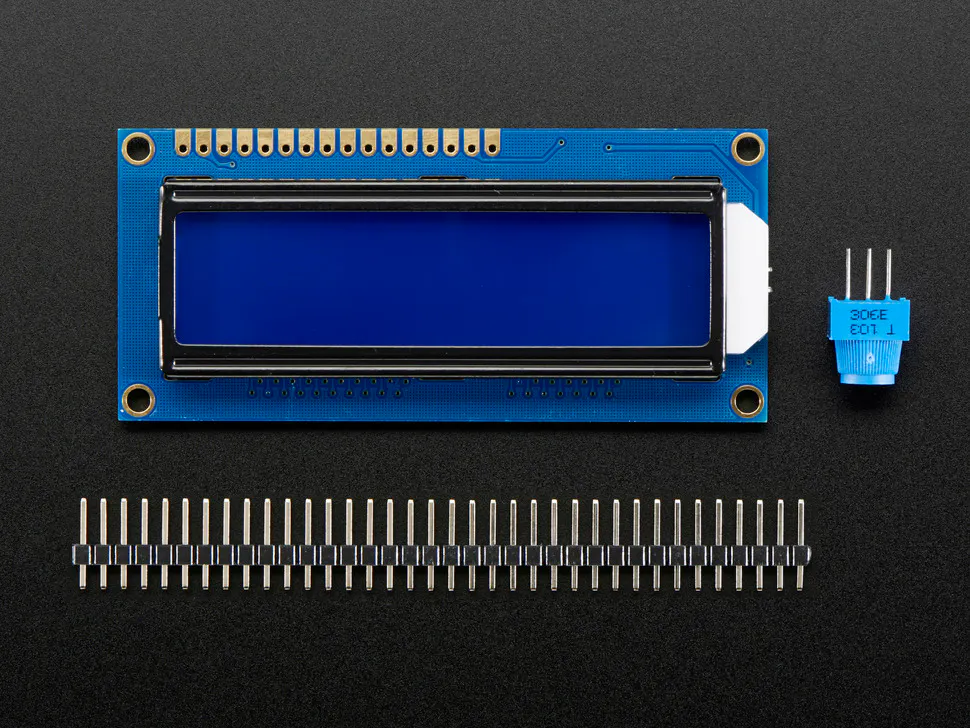Adafruit Standard LCD - 16x2 White on Blue
×1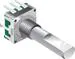Rotary Encoder with Push-Button
×1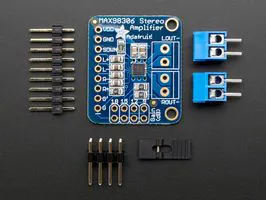Development Board, Class D Audio Amplifier Module
×1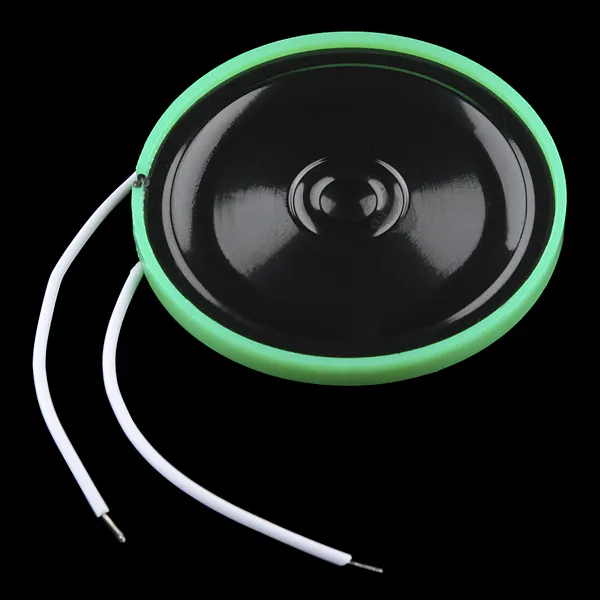Speaker: 0.25W, 8 ohms
×1
 ferite rod from old MW radio
×1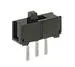Slide Switch
×1

### Software apps and online servicesArduino IDE

### Hand tools and fabrication machinesSoldering iron (generic)

## Code

### Code

C/C++
```/**************************************************************************************************
JCR Si4730/5 Receiver LW/MW/SW/FM - Version to share. See schematics for wiring details.
Written by Julio C. Rosa - The CesarSound - May/2019

Commands:
RSQMetrics rsq; radio.getRSQ(&rsq); (rsq.stereo); print_number(rsq.stereoBlend); print_number(rsq.SNR);
print_mode(); print_station_freq(); print_volume(); print_mute(); print_home();
Serial.write(programType); (rds1); (rds2); (rds3); (rds4); (rds5);

*****************************************************************************************************/

// Library
#include <Si4735.h>
#include <LiquidCrystal.h>
#include <rotary.h>
#include "Wire.h"

// I/O Settings
Rotary r = Rotary(2, 3);
LiquidCrystal lcd(12, 13, 7, 6, 5, 4);

const byte band = A0;

// Variable setting
unsigned int freq = 880;
unsigned int freqmax = 10800;
unsigned int freqmin = 50;
unsigned int fstep = 10;
unsigned int freqold;
unsigned int buttonstate;
unsigned int count = 15;

// Create an instance of the Si4730/5 named radio.

void setup() {
lcd.begin(16, 2);

PCICR |= (1 << PCIE2);
PCMSK2 |= (1 << PCINT18) | (1 << PCINT19);
sei();

//Pushbutton seletor de bandas
pinMode(band, INPUT_PULLUP);

// Initialize the radio. Possible modes are AM, FM, SW, LW.

command_am();

// Set the initial Frequency

lcd.setCursor(0, 2);
lcd.print("JCR Multiband Rx");

lcd.setCursor(0, 0);
lcd.print("F>>");
lcd.setCursor(3, 0);
lcd.print(":");
lcd.setCursor(13, 0);
lcd.print("kHz");
}

// Encoder Interrupt
ISR(PCINT2_vect) {
unsigned char result = r.process();
if (result) {
if (result == DIR_CW) {
freq = freq + fstep;
if (freq >= freqmax) {
freq = freqmax;
}
}
else {
freq = freq - fstep;
if (freq <= freqmin) {
freq = freqmin;
}
}
}
}

void loop() {
if (freqold != freq) {
tune();
}
freqold = freq;

lcd.setCursor(5, 0);
lcd.print(freq);
lcd.print("  ");

buttonstate = digitalRead(band);  // Seletor de bandas
if (buttonstate == LOW) {
inc_preset();
}

// Medidor - S-Meter (dBu) e SNR (dB) - uncomment if want to use
RSQMetrics rsq;
lcd.setCursor(0, 2);
lcd.print("dBu: ");
lcd.setCursor(9, 2);
lcd.print("dB: ");
lcd.print(rsq.SNR);
}

// Presets INC
void inc_preset() {
count++;
if (count > 15)
count = 1;
bandpresets();
delay(100);
}

// Presets Seletor de Bandas
void bandpresets() {

lcd.setCursor(0, 0);
switch (count)  {
case 1:
freq = 370;
command_am();
fstep = 5;
lcd.print("LW1");
break;
case 2:
freq = 620;
fstep = 5;
lcd.print("MW1");
break;
case 3:
freq = 840;
fstep = 5;
lcd.print("MW2");
break;
case 4:
freq = 3700;
fstep = 5;
lcd.print("SW0");
break;
case 5:
freq = 4985;
fstep = 5;
lcd.print("SW1");
break;
case 6:
freq = 6010;
fstep = 1;
lcd.print("SW2");
break;
case 7:
freq = 7200;
fstep = 1;
lcd.print("SW3");
break;
case 8:
freq = 10000;
fstep = 5;
lcd.print("SW4");
break;
case 9:
freq = 11940;
fstep = 5;
lcd.print("SW5");
break;
case 10:
freq = 13710;
fstep = 5;
lcd.print("SW6");
break;
case 11:
freq = 15400;
fstep = 5;
lcd.print("SW7");
break;
case 12:
freq = 17560;
fstep = 5;
lcd.print("SW8");
break;
case 13:
freq = 21505;
fstep = 5;
lcd.print("SW9");
break;
case 14:
freq = 27015;
fstep = 5;
lcd.print("CB1");
break;
case 15:
freq = 9700;
fstep = 10;
lcd.print("FM1");
break;
}
}

void tune() {
}

//Commands soft mute off / AVC max gain
void command_am() {
radio.setProperty(PROP_AM_SOFT_MUTE_MAX_ATTENUATION, 0); // 0 (OFF) - 63
radio.setProperty(PROP_AM_AUTOMATIC_VOLUME_CONTROL_MAX_GAIN, 0x3A98); // 1000 - 7800
}
```

### Libraries

C/C++
`No preview (download only).`

## Credits

### Mirko Pavleski

21 projects • 549 followers